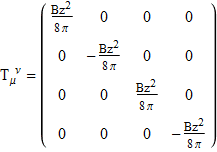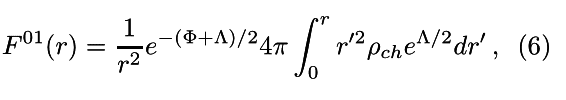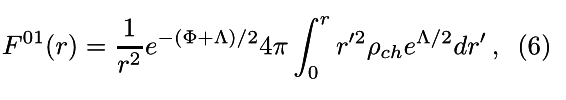# Construct electromagnetic stress-energy tensor for a non-flat metric

Hi, I am having problems in constructing a stress-energy tensor representing a constant magnetic field Bz in the $\hat{z}$ direction. The coordinate system is a cylindric {t,r,z,$\varphi$}. The metric signature is (+,-,-,-).

I ended with the following mixed stress-energy tensor:Is this correct?

How to properly construc this general magnetic field stress-energy tensor that exists without sources?

Thank you,

Coleman

#### Attachments

Starting fro the field tensor F the emt is

##T^{\mu\nu} = \frac{1}{\mu_0}[ F^{\mu \alpha}F_{\alpha}{}^{\nu} - \frac{1}{4} \eta^{\mu\nu}F_{\alpha\beta}F^{\alpha\beta}]##

You could replace the flat space metric ##\eta^{\mu\nu}## with the general ##g^{\mu\nu}## but I'm not sure if it would be meaningful because the measurements are always in a local frame.

Last edited:
Right, but what would be the form of the emt F? I know that there will be some metric functions inside (the metric is not flat), but I don't know how to construct it. From F I can calculate the stress-energy tensor.

Thank you

Right, but what would be the form of the emt F? I know that there will be some metric functions inside (the metric is not flat), but I don't know how to construct it. From F I can calculate the stress-energy tensor.

Thank you
The definition of F is ##F_{\mu\nu}=\nabla_\mu A_\nu-\nabla_\nu A_\mu=\partial_\mu A_\nu-\partial_\nu A_\mu## so it appears that that F is the same in flat and curved spacetime.

(caution - I fixed a typo in my earlier post )

Last edited:
Are you sure? Look for example at this paper: http://arxiv.org/pdf/0907.5537v2

We can see at equation 6 that the electromagnetic tensor has functions of the metric that are to be found.

There they use the Maxwell equations to find this tensor, but since I have no charge or currents, I want to know if there's some direct way to construct the electromagnetic tensor for a constant magnetic field and consequently find the mixed stress-energy tensor.

If I use a electromanetic tensor without the potential functions of the metric, then my mixed stress-energy tensor still contains those potentials, and from what I know, mixed stress-energy tensors are free of them.

Are you sure? Look for example at this paper: http://arxiv.org/pdf/0907.5537v2

We can see at equation 6 that the electromagnetic tensor has functions of the metric that are to be found.

There they use the Maxwell equations to find this tensor, but since I have no charge or currents, I want to know if there's some direct way to construct the electromagnetic tensor for a constant magnetic field and consequently find the mixed stress-energy tensor.

If I use a electromanetic tensor without the potential functions of the metric, then my mixed stress-energy tensor still contains those potentials, and from what I know, mixed stress-energy tensors are free of them.
Do you mean equation 4 ? That is equivalent to the one I gave.

The problem with mixed tensors is that they are unphysical. For instance T00 can be negative.

If you are given the field tensor then you can always calculate the emt, then if you want the mixed tensor you can always raise or lower an index of the covariant or contravariant T.

##{T^\mu}_\nu= g_{\nu\rho}T^{\mu\rho}=g^{\mu\rho}T_{\nu\rho}##

Last edited:
WannabeNewton
You say you have the ##B## field but the ##B## field only has meaning relative to a given observer. At any given event ##p##, if ##u^{\mu}## is the 4-velocity of an observer ##O## at ##p## then the EM field tensor can be decomposed at ##p## as ##F_{\mu\nu} = 2E_{[\mu}u_{\nu]} + \epsilon_{\mu\nu\alpha\beta}u^{\alpha} B^{\beta}## where ##E^{\mu}, B^{\mu}## are the electric and magnetic fields relative to ##O##.

I mean equation 6, the electromagnetic tensor defined there has only two components, F_01 and F_10:You can see that it has the metric potentials inside: $\Phi$ and $\Lambda$

#### Attachments

I mean equation 6, the electromagnetic tensor defined there has only two components, F_01 and F_10:You can see that it has the metric potentials inside: $\Phi$ and $\Lambda$
Do you mean the vector potentials ( ie components of Aμ) ?

Do you mean the vector potentials ( ie components of Aμ) ?

No, the electromagnetic tensor F -> F_01 and F_10 components.

No, the electromagnetic tensor F -> F_01 and F_10 components.
OK, I saw the way the metric is written. I think the metric coefficients are there to raise or lower indexes, but I have not fully understood where it all comes from. I have run out of time, I'm sorry if my replies have not helped.

Ok Mentz, thanks for the help. Have a nice day

PeterDonis
Mentor
Are you sure?

Mentz114 is correct, the connection coefficient terms cancel because ##F_{\mu \nu}## is antisymmetric and the connection coefficients are symmetric, so covariant derivatives can be replaced by partial derivatives. So ##F_{\mu \nu}##, with two lower indexes, does not contain any metric coefficients.

However, in order to get the stress-energy tensor from ##F_{\mu \nu}##, you have to raise some of the indexes, and that brings in the metric coefficients. For a pure magnetic field in the ##z## direction, we will have ##F_{12} = - F_{21} = B## as the only nonzero components of the EM field tensor (note that this is in Cartesian coordinates, not cylindrical; I haven't worked out the cylindrical case, but it should be straightforward to transform into it from Cartesian). (Note that this is different from the EMT in the paper you linked to because that paper is analyzing a different scenario, a spherically symmetric charged object.) So the SET will be, using Mentz114's formula but with the general curved metric ##g_{\mu \nu}##:

$$T^{\mu \nu} = F^{\mu \alpha} F_{\alpha}{}^{\nu} - \frac{1}{4} g^{\mu \nu} F_{\alpha \beta} F^{\alpha \beta}$$

Since we only know ##F## with two lower indexes, we have to contract with the metric to raise them where necessary:

$$T^{\mu \nu} = g^{\mu \rho} g^{\alpha \sigma} F_{\rho \sigma} g^{\lambda \nu} F_{\alpha \lambda} - \frac{1}{4} g^{\mu \nu} F_{\alpha \beta} g^{\alpha \rho} g^{\beta \sigma} F_{\rho \sigma}$$

Now ##F## appears everywhere with lower indexes, which is what we have a formula for from above; but you can also see that the metric coefficients appear a number of times. To fully solve this you would then have to write out the Einstein tensor, set it equal to ##8 \pi## times the SET written above, and use the known components of ##F## to derive differential equations for the metric coefficients.